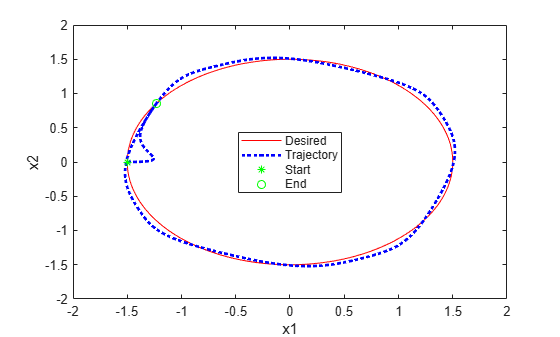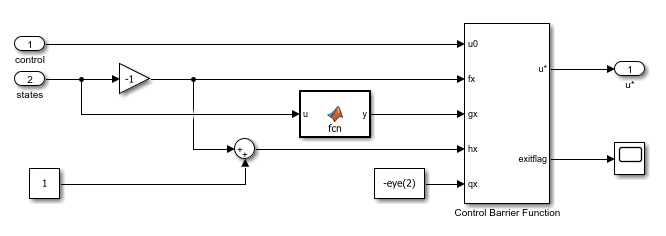# Enforce Barrier Certificate Constraints for PID Controllers

This example shows how to enforce barrier certificate constraints for a PID controller application using the Barrier Certificate Enforcement block.

### Overview

For this example, the plant dynamics are described by the following equations .

`${\stackrel{˙}{\mathit{x}}}_{1}=-{\mathit{x}}_{1}+\left({\mathit{x}}_{1}^{2}+1\right){\mathit{u}}_{1}$`

`${\stackrel{˙}{\mathit{x}}}_{2}=-{\mathit{x}}_{2}+\left({\mathit{x}}_{2}^{2}+1\right){\mathit{u}}_{2}$`

The goal for the plant is to track desired trajectories, defined as:

`$\stackrel{˙}{\theta }=0.1\pi$`

`${\stackrel{˙}{\mathit{x}}}_{1\mathit{d}}=-\mathit{r}\text{\hspace{0.17em}}\mathrm{cos}\left(\theta \right)$`

`${\stackrel{˙}{\mathit{x}}}_{2\mathit{d}}=\mathit{r}\text{\hspace{0.17em}}\mathrm{sin}\left(\theta \right)$`

For an example that applies a predicted constraint function to the same PID control application, see Enforce Constraints for PID Controllers.

Configure model parameters and initial conditions.

```r = 1.5; % Radius for desired trajectory Ts = 0.1; % Sample time Tf = 22; % Duration x0_1 = -r; % Initial condition for x1 x0_2 = 0; % Initial condition for x2```

### Design PID Controllers

Before applying constraints, design PID controllers for tracking the reference trajectories. The `barrierCertificatePID` model contains two PID controllers with tuned gains. For more information on tuning PID controllers in Simulink® models, see Introduction to Model-Based PID Tuning in Simulink.

```constrained = 0; % Disable barrier certificate constraint enforcement mdl = 'barrierCertificatePID'; open_system(mdl)```

Simulate the PID controllers and plot their tracking performance.

```% Simulate the model. out = sim(mdl); % Extract trajectories. logData = out.logsout; x1_traj = zeros(size(out.tout)); x2_traj = zeros(size(out.tout)); for ct = 1:size(out.tout,1) x1_traj(ct) = logData{3}.Values.Data(:,:,ct); x2_traj(ct) = logData{4}.Values.Data(:,:,ct); end x1_des = logData{1}.Values.Data; x2_des = logData{2}.Values.Data; % Plot trajectories. figure('Name','Tracking with Constraint'); plot(x1_des,x2_des,'r') xlabel('x1') ylabel('x2') hold on plot(x1_traj,x2_traj,'b:','LineWidth',2) hold on plot(x1_traj(1),x2_traj(1),'g*') hold on plot(x1_traj(end),x2_traj(end),'go') legend('Desired','Trajectory','Start','End','Location','best')```### Barrier Certificates

For this example, you enforce barrier certificate constraints for the same plant model and PID controllers.

The feasible region for the plant is given by $\left\{\mathit{x}:{\mathit{x}}_{1}\le 1,{\mathit{x}}_{2}\le 1\right\}$. Therefore, the barrier certificates are given by $\mathit{h}\left(\mathit{x}\right)=\left[\begin{array}{c}1-{\mathit{x}}_{1}\\ 1-{\mathit{x}}_{2}\end{array}\right]\ge 0$.

The partial derivative of $\mathit{h}\left(\mathit{x}\right)$ over $\mathit{x}$ is given by $\mathit{q}\left(\mathit{x}\right)=$$\left[\begin{array}{cc}-1& 0\\ 0& -1\end{array}\right]$.

The Barrier Certificate Enforcement block accepts plant dynamics in the form $\stackrel{˙}{\mathit{x}}=\mathit{f}\left(\mathit{x}\right)+\mathit{g}\left(\mathit{x}\right)\mathit{u}$. For this application, $\mathit{f}\left(\mathit{x}\right)=\left[\begin{array}{c}-{\mathit{x}}_{1}\\ -{\mathit{x}}_{2}\end{array}\right]$ and $\mathit{g}\left(\mathit{x}\right)=\left[\begin{array}{cc}{\mathit{x}}_{1}^{2}+1& 0\\ 0& {\mathit{x}}_{2}^{2}+1\end{array}\right]$.

### Simulate PID Controller with Barrier Certificate Constraint

To view the constraint implementation, open the `Constraint` > `Constrained` subsystem.

Enable barrier certificate constraint enforcement.

`constrained = 1;`Run the model and plot the simulation results. The plot shows that the plant states are less than one.

```% Simulate the model. out = sim(mdl); % Extract trajectories. logData = out.logsout; x1_traj = zeros(size(out.tout)); x2_traj = zeros(size(out.tout)); for ct = 1:size(out.tout,1) x1_traj(ct) = logData{3}.Values.Data(:,:,ct); x2_traj(ct) = logData{4}.Values.Data(:,:,ct); end x1_des = logData{1}.Values.Data; x2_des = logData{2}.Values.Data; % Plot trajectories. figure('Name','Tracking with Constraint'); plot(x1_des,x2_des,'r') xlabel('x1') ylabel('x2') hold on plot(x1_traj,x2_traj,'b:','LineWidth',2) hold on plot(x1_traj(1),x2_traj(1),'g*') hold on plot(x1_traj(end),x2_traj(end),'go') legend('Desired','Trajectory','Start','End','Location','best')```The Barrier Certificate Enforcement block successfully constrains the control actions such that the plant states remain less than one.

Close the model.

`bdclose(mdl)`

### References

 Robey, Alexander, Haimin Hu, Lars Lindemann, Hanwen Zhang, Dimos V. Dimarogonas, Stephen Tu, and Nikolai Matni. "Learning Control Barrier Functions from Expert Demonstrations." Preprint, submitted April 7, 2020. https://arxiv.org/abs/2004.03315